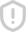### latex中的各种特殊符号 评分Latex中的各种特殊字符：\alpha θ \theta o o τ \tau β \beta ϑ \vartheta π \pi υ \upsilon γ \gamma ι \iota \$ \varpi φ \phi δ \delta κ \kappa ρ \rho ϕ \varphi \epsilon λ \lambda % \varrho χ \chi ε \varepsilon µ \mu σ \sigma ψ \psi ζ \zeta ν \nu ς \varsigma ω \omega η \eta ξ \xi Γ \Gamma Λ \Lambda Σ \Sigma Ψ \Ps举报收藏分享